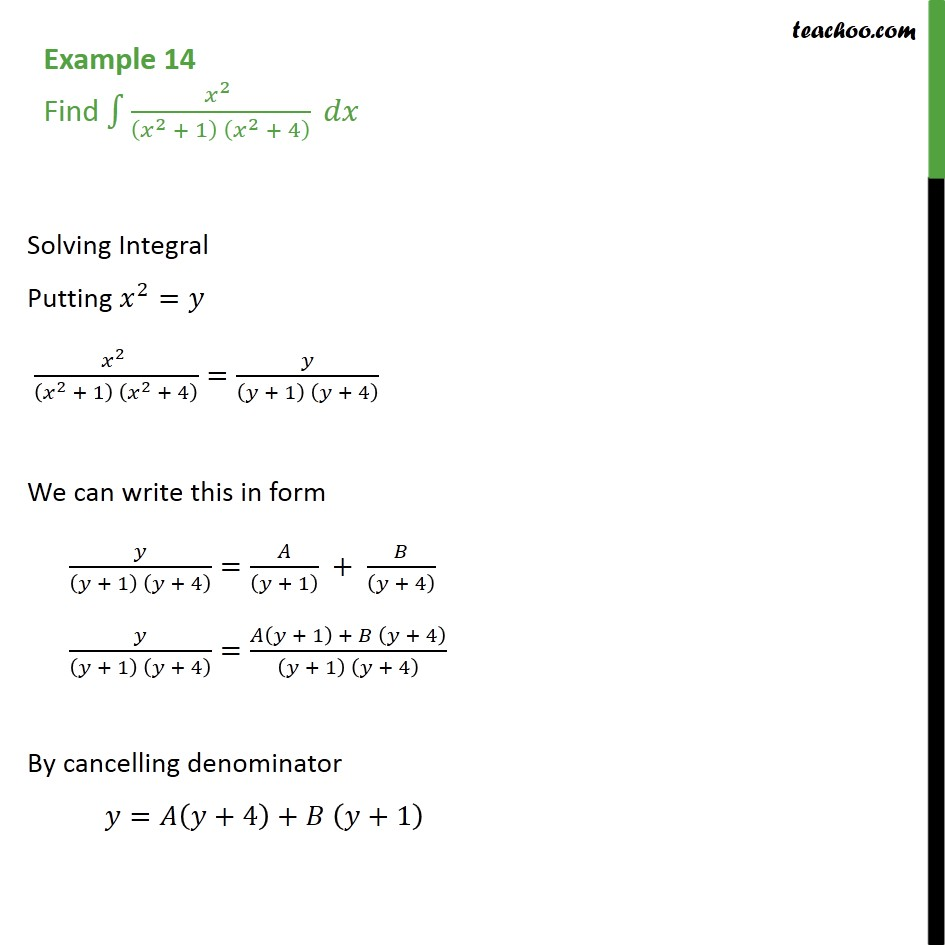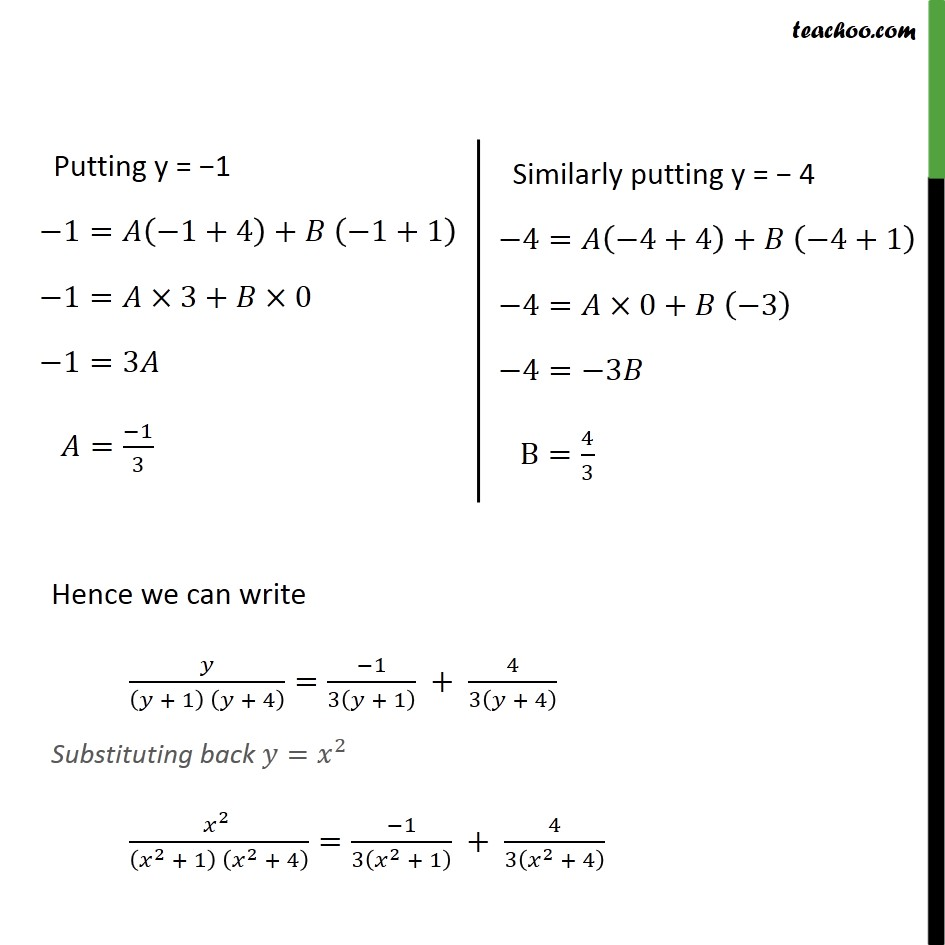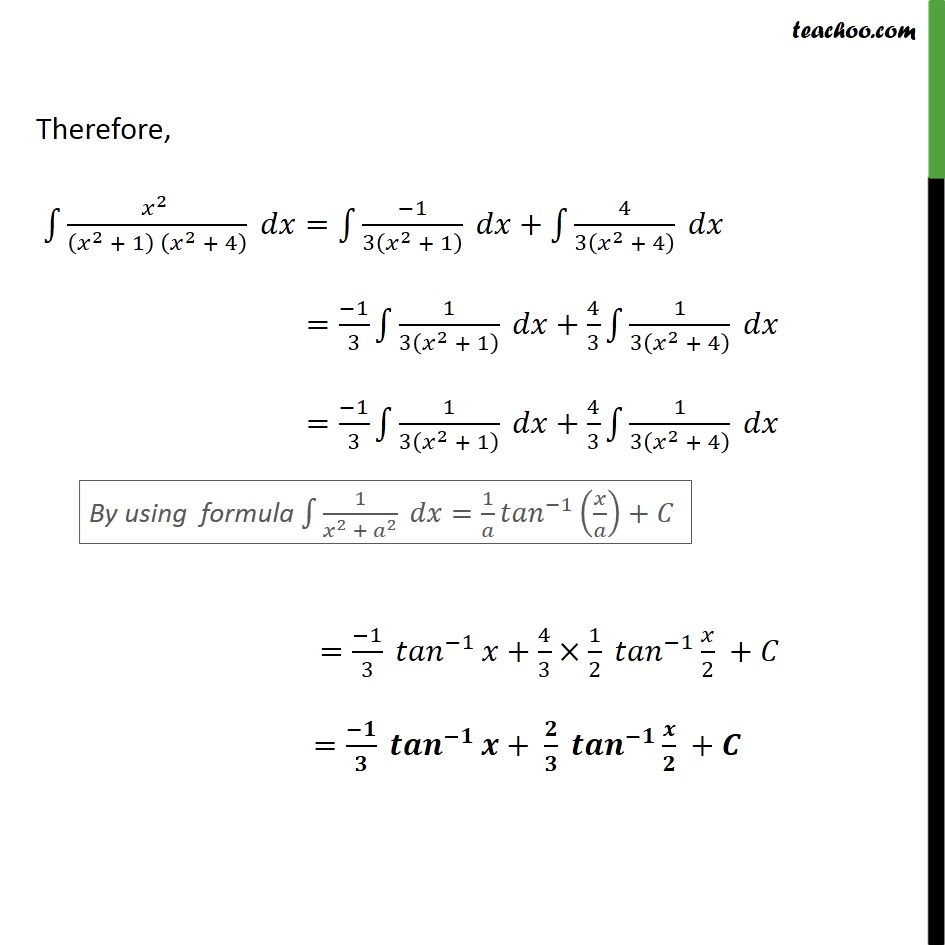Integration by partial fraction - Type 1

Chapter 7 Class 12 Integrals
Concept wiseIntroducing your new favourite teacher - Teachoo Black, at only ₹83 per month

### Transcript

Example 14 Find 2 2 + 1 2 + 4 Solving Integral Putting 2 = 2 2 + 1 2 + 4 = + 1 + 4 We can write this in form + 1 + 4 = + 1 + + 4 + 1 + 4 = + 1 + + 4 + 1 + 4 By cancelling denominator = +4 + +1 = +4 + +1 Hence we can write + 1 + 4 = 1 3 + 1 + 4 3 + 4 Substituting back = 2 2 2 + 1 2 + 4 = 1 3 2 + 1 + 4 3 2 + 4 Therefore, 2 2 + 1 2 + 4 = 1 3 2 + 1 + 4 3 2 + 4 = 1 3 1 3 2 + 1 + 4 3 1 3 2 + 4 = 1 3 1 3 2 + 1 + 4 3 1 3 2 + 4 = 1 3 1 + 4 3 1 2 1 2 + = + +